# Acetic acid has a Ka of 1.8*10^-5. Three acetic acid/ acetate buffer solutions, A,B, and C, wer made using varying conce...

Acetic acid has a Ka of 1.8*10^-5. Three acetic acid/ acetate buffer solutions, A,B, and C, wer made using varying concentrations: 1. [acetic acid] ten times greater than [acetate] 2. [acetate] ten times greater than [acetic acid] 3. [acetate] = [acetic acid] Match each buffer to the expected pH pH = 3.74 pH= 4.74 pH = 5.74

Concepts and reason

The concept used to solve this question is to match the ${\rm{pH}}$ with the corresponding buffer solutions A, B and C.

Fundamentals

$pH$ is the measure of hydrogen ion concentration. Lower the $pH$ , higher is the hydrogen ion concentration and lower is the hydroxide ion concentration.

An equilibrium constant for the dissociation reaction is the equation expressing the extent of dissociation into ions which is equal to the product of the concentrations of the respective ions divided by the concentration of the undissociated molecule.

Consider a dissociation reaction of acetic acid, ${\rm{C}}{{\rm{H}}_3}{\rm{COOH}} + {{\rm{H}}_2}{\rm{O}}\longrightarrow{{}}{{\rm{H}}_3}{{\rm{O}}^ + } + {\rm{C}}{{\rm{H}}_3}{\rm{CO}}{{\rm{O}}^ - }$ .

The equilibrium constant for the dissociation of ${\rm{HA}}$ is given as follows:

${K_a} = \frac{{\left[ {{{\rm{H}}_3}{{\rm{O}}^ + }} \right]\left[ {{\rm{C}}{{\rm{H}}_3}{\rm{CO}}{{\rm{O}}^ - }} \right]}}{{\left[ {{\rm{C}}{{\rm{H}}_3}{\rm{COOH}}} \right]}}$

$p{K_a}$ is also the measure of acidic strength.

The formula relating ${K_a}$ and $p{K_a}$ is as follows:

$p{K_a} = - \log {K_a}$

The Henderson-Hasselbalch equation to calculate the ${\rm{pH}}$ of a buffer solution is as follows:

${\rm{pH}} = p{K_a} + \log \frac{{\left[ {{\rm{C}}{{\rm{H}}_3}{\rm{CO}}{{\rm{O}}^ - }} \right]}}{{\left[ {{\rm{C}}{{\rm{H}}_3}{\rm{COOH}}} \right]}}$

Given, ${K_a} = 1.8 \times {10^{ - 5}}$

The $p{K_a}$ of the acid is calculated as follows:

$\begin{array}{l}\\p{K_a} = - \log \left( {1.8 \times {{10}^{ - 5}}} \right)\\\\{\rm{ = 4}}{\rm{.74}}\\\end{array}$

Given that, for buffer A,

$\left[ {{\rm{Acetic acid}}} \right]$ is 10 times greater the concentration of $\left[ {{\rm{Acetate}}} \right]$ .

So,

$\begin{array}{l}\\\left[ {{\rm{Acetic acid}}} \right] = 10x\\\\\left[ {{\rm{Acetate}}} \right] = x\\\end{array}$

Substitute the values in the Henderson-Hasselbalch equation and calculate the ${\rm{pH}}$ of the buffer as follows:

$\begin{array}{l}\\{\rm{pH}} = 4.74 + \log \frac{x}{{{\rm{10}}x}}\\\\{\rm{ = 3}}{\rm{.74}}\\\end{array}$

Given that, for buffer B,

$\left[ {{\rm{Acetate}}} \right]$ is 10 times greater the concentration of $\left[ {{\rm{Acetic acid}}} \right]$ .

So,

$\begin{array}{l}\\\left[ {{\rm{Acetic acid}}} \right] = x\\\\\left[ {{\rm{Acetate}}} \right] = 10x\\\end{array}$

Substitute the values in the Henderson-Hasselbalch equation and calculate the ${\rm{pH}}$ of the buffer as follows:

$\begin{array}{l}\\{\rm{pH}} = 4.74 + \log \frac{{10x}}{x}\\\\{\rm{ = 5}}{\rm{.74}}\\\end{array}$

Given that, for buffer C,

$\left[ {{\rm{Acetic acid}}} \right] = \left[ {{\rm{Acetate}}} \right]$ .

So,

$\begin{array}{l}\\\left[ {{\rm{Acetic acid}}} \right] = x\\\\\left[ {{\rm{Acetate}}} \right] = x\\\end{array}$

Substitute the values in the Henderson-Hasselbalch equation and calculate the ${\rm{pH}}$ of the buffer as follows:

$\begin{array}{l}\\{\rm{pH}} = 4.74 + \log \frac{x}{x}\\\\{\rm{ = 4}}{\rm{.74}}\\\end{array}$

Ans:

The ${\rm{pH}}$ of the three buffer solutions are as follows:

$\begin{array}{l}\\{\rm{Buffer A}}:3.74\\\\{\rm{Buffer B}}:5.74\\\\{\rm{Buffer C}}:4.74\\\end{array}$

##### Add Answer of: Acetic acid has a Ka of 1.8*10^-5. Three acetic acid/ acetate buffer solutions, A,B, and C, wer made using varying conce...
Similar Homework Help Questions
• ### Part A Acetic acid has a Ka of 1.8×10−5. Three acetic acid/acetate buffer solutions, A, B, and C, were made using varyi...

Part A Acetic acid has a Ka of 1.8×10−5. Three acetic acid/acetate buffer solutions, A, B, and C, were made using varying concentrations: [acetic acid] ten times greater than [acetate], [acetate] ten times greater than [acetic acid], and [acetate]=[acetic acid]. Match each buffer to the expected pH. Drag each item to the appropriate bin. Part B How many grams of dry NH4Cl need to be added to 2.40 L of a 0.100 M solution of ammonia, NH3, to prepare a...

• ### Help! Will Give high Rating For Sure!!

Just as is the negative logarithm of, is the negative logarithm of ,The Henderson-Hasselbalch equation is used to calculate the of buffer solutions:Notice that the of a buffer has a valueclose to the of theacid, differing only by the logarithm of theconcentration ratio .Part AAcetic acid has a of . Three acetic acid/acetate buffer solutions, A, B,and C, were madeusing varying concentrations: ten times greaterthan , ten times greater than, and.Match each buffer to the expected .1 .pH= 3.742. pH=4.743....

• ### (C))3.75 moo (D) 4.75 (E) 5.75 bios nA oir 9. Ka of acetic acid is 1.8 x 10. What is the pH of a solution 0.1M acet...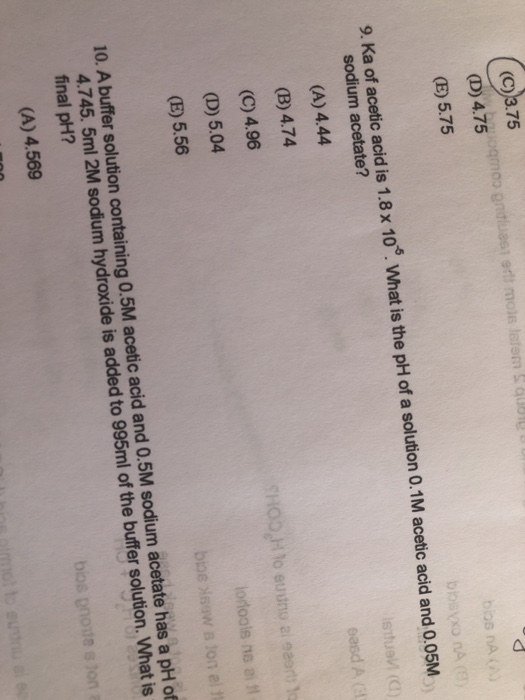(C))3.75 moo (D) 4.75 (E) 5.75 bios nA oir 9. Ka of acetic acid is 1.8 x 10. What is the pH of a solution 0.1M acetic acid and 0.05M sodium acetate? (A) 4.44 oasd A (B) 4.74 (C) 4.96 (D) 5.04 (E) 5.56 10. A buffer solution containing 0.5M acetic acid and 0.5M sodium acetate hais a pH ot 4.745. 5ml 2M sodium hydroxide is added to 995ml of the buffer solution. What is final pH? (A) 4.569 (C))3.75...

• ### 2) A buffer solution is prepared by dissolving Sodium Acetate and Acetic acid solutions. If the...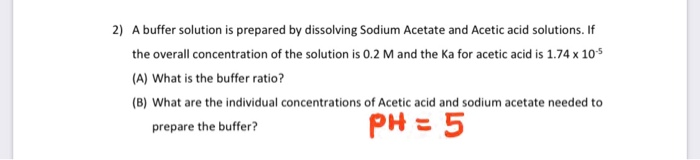2) A buffer solution is prepared by dissolving Sodium Acetate and Acetic acid solutions. If the overall concentration of the solution is 0.2 M and the Ka for acetic acid is 1.74 x 105 (A) What is the buffer ratio? (B) What are the individual concentrations of Acetic acid and sodium acetate needed to prepare the buffer? PH = 5 Can you please write down any assumptions needed for this particular problem? My Professor needs to see thought process. Thank...

• ### A buffer solution is made up of equal volumes of 0.100M acetic acid and 0.500M sodium acetate

A buffer solution is made up of equal volumes of 0.100M acetic acid and 0.500M sodium acetate. Ka for the acetic acid=1.8 x 10^-5a)What is the pH of the buffer?b)Assuminmg no change in volume, what is the pH of the solution after the addtion of 0.01 moles of H+ is added?c) Assuming no change in volume, what would the pH of the solution be is 0.01 moles of OH- were added instead of 0.01 M H+?

• ### a buffer solution of pH =5.30 can be prepared by dissolving acetic acid and sodium acetate...

a buffer solution of pH =5.30 can be prepared by dissolving acetic acid and sodium acetate in water. How many moles of sodium acetate must be added to 1 L of 0.25 M acetic acid to prepare the buffer? Ka(CH3COOH)=1.8 x 10^-5

• ### A buffer solution is 0.78 M in acetic acid and 0.22 M in sodium acetate. Calculate...

A buffer solution is 0.78 M in acetic acid and 0.22 M in sodium acetate. Calculate the solution pH after adding 0.80 g of solid NaOH to 100.0 mL of the buffer solution. Ka of acetic acid is 1.8  10−5 . Assume negligible volume change.

• ### With this information what is the PH of the .1 M acetic acid sol and .1...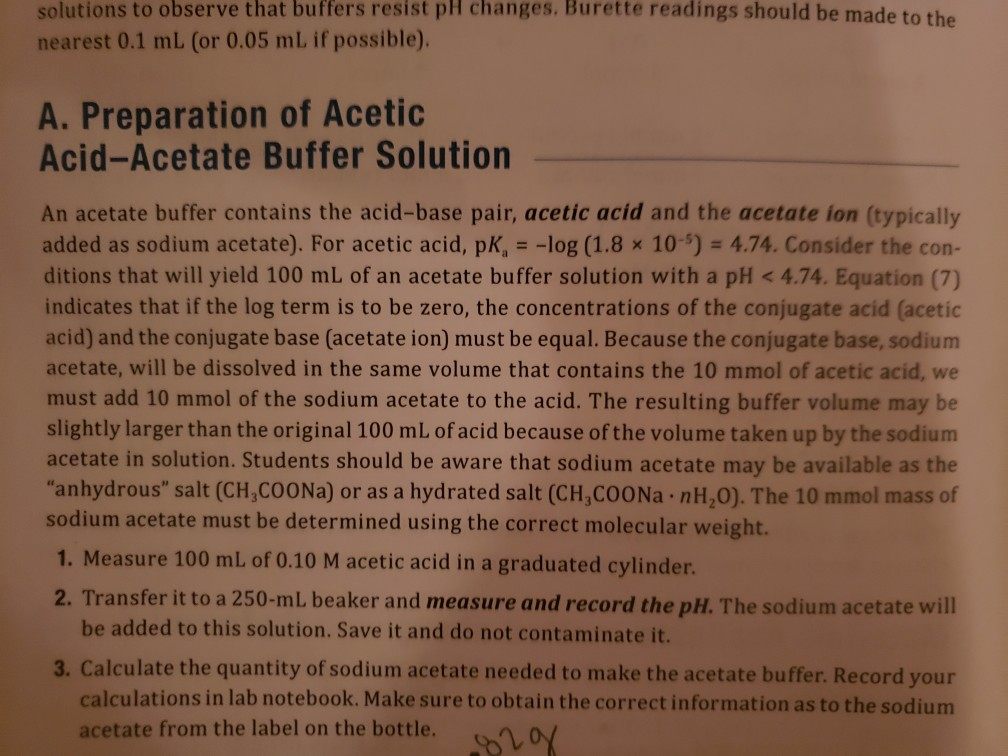With this information what is the PH of the .1 M acetic acid sol and .1 M acetic acid buffer sol? Please help solutions to observe that buffers resist pH changes. Burette readings should be made to the nearest 0.1 mL (or 0.05 mL if possible), A. Preparation of Acetic Acid-Acetate Buffer Solution An acetate buffer contains the acid-base pair, acetic acid and the acetate ion (typically added as sodium acetate). For acetic acid, pK, = -log (1.8 x 10-)...

• ### Calculate the pH of an acetic acid/acetate buffer in which the concentration of acetic acid is always 0.21 M, but...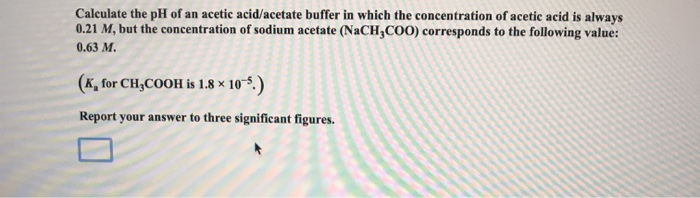Calculate the pH of an acetic acid/acetate buffer in which the concentration of acetic acid is always 0.21 M, but the concentration of sodium acetate (NaCH,COO) corresponds to the following value: 0.63 M. (K, for CH,COOH is 1.8 x 10 5.) Report your answer to three significant figures.

• ### A buffer containing acetic acid and sodium acetate has a pH of 5.55. The K, value...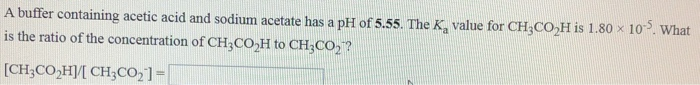A buffer containing acetic acid and sodium acetate has a pH of 5.55. The K, value for CH3CO,H is 1.80 x 10. What is the ratio of the concentration of CH3CO H to CH3CO,t? [CH,CO,H1 CH2C0, 1 = Calculate the pH of a solution that has an ammonium chloride concentration of 0.054 M and an ammonia concentration of 0.053 M. Kb = 1.8 x 10-5 pH = What is the pH of 0.35 M acetic acid to 1.00 L of...

Need Online Homework Help?